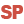HelpWLanguageWLanguage functionsStandard functionsEncryption/compression functionsLuhnCalculatePresentationExampleSee alsoCompressCompression / encryption functionsConstants for encryption/compressionDecodeDecrypt (Example)DecryptDecryptAsymmetricDecryptAsymmetricEnvelopedDecryptStandard (Example)DecryptStandardEncodeEncrypt (Example)EncryptEncryptAsymmetricEncryptAsymmetricEnvelopedEncryptGenerateRSAKeyEncryptStandard (Example)EncryptStandardLuhnCalculateLuhnCheckUUDecodeUUEncodeUncompress
 WINDEVWEBDEVWINDEV MobileOthersLuhnCalculate (Function) In french: LuhnCalcule Calculates the next Luhn check digit for a given sequence. Example sNumberToCheck is string = "120 027 016 0056"cCheckDigit is character = LuhnCalculate(sNumberToCheck)Info("The check digit is [%cCheckDigit%]") Syntax = LuhnCalculate() : Character stringLuhn check digit for the given sequence : Character stringSequence from which the Luhn digit will be determined. This sequence must be composed of numbers and spaces only (spaces are not relevant for the formula).Business / UI classification: Neutral codeComponent: wd280std.dll Minimum version required Version 27 This page is also available for…